# My textbook is deriving wave speed on a string under tension

• I
• annamal
In summary, the textbook's derivation of wave speed on a string under tension assumes that the theta angle between the forces is the angle between the x and y positions on the string, which is not always the case.

#### annamal

My textbook is deriving wave speed on a string under tension with confusing thetas. It assumes ##\tan \theta_1 = \frac{-F_1}{F_T}## and ##\tan \theta_2 = \frac{F_2}{F_T}## which confuses me. I know for sure theta is the angle due to the position of y and x, ##\tan \theta = \frac{y}{x}##, but I am confused how you can assume that theta is the angle between the forces. Attached is an image of a graph of the y position vs x position of a wave.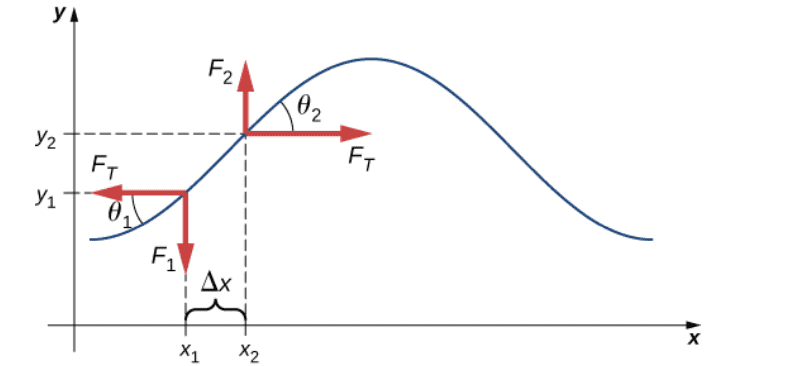annamal said:
My textbook is deriving wave speed on a string under tension with confusing thetas. It assumes ##\tan \theta_1 = \frac{-F_1}{F_T}## and ##\tan \theta_2 = \frac{F_2}{F_T}## which confuses me. I know for sure theta is the angle due to the position of y and x, ##\tan \theta = \frac{y}{x}##, but I am confused how you can assume that theta is the angle between the forces. Attached is an image of a graph of the y position vs x position of a wave.
View attachment 299804
Consider point 2. Draw in the force ##F## exerted on the small segment of string. What are the x and y components of that force in terms of ##F## and ##\theta_2##?

vela said:
Consider point 2. Draw in the force ##F## exerted on the small segment of string. What are the x and y components of that force in terms of ##F## and ##\theta_2##?
That's not answering my question of how come ##\theta_2## and ##\theta_1## are the angles between the forces. ##\theta_2## could be greater or smaller. ##\theta_2## is the angle due to the y and x quantities but not necessarily the angle for the Fx and Fy quantities.

OK, let's try a different tack.

Why do you think ##\tan \theta = \frac yx##? I don't see where you're getting that idea from the diagram you provided.

Also, what you do mean by ##\theta## being the angle between the forces? The angle between the components shown is 90 degrees.

vela said:
OK, let's try a different tack.

Why do you think ##\tan \theta = \frac yx##? I don't see where you're getting that idea from the diagram you provided.

Also, what you do mean by ##\theta## being the angle between the forces? The angle between the components shown is 90 degrees.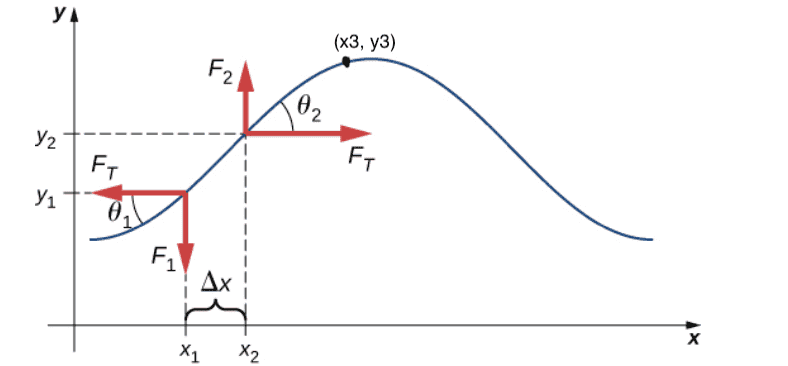In the image attached, I added a point (x3, y3). ##\tan(\theta_2) = \frac{y3 - y2}{x3 - x2}## which has nothing to do with the forces. The forces may have a different ##\theta## where ##\tan(\theta) = \frac{Fy}{Fx}##

Which way do you think the force the righthand portion of the string exerts on the segment points? (That's why I asked you to draw in that force.) Do you think the situation I drew in the picture is possible?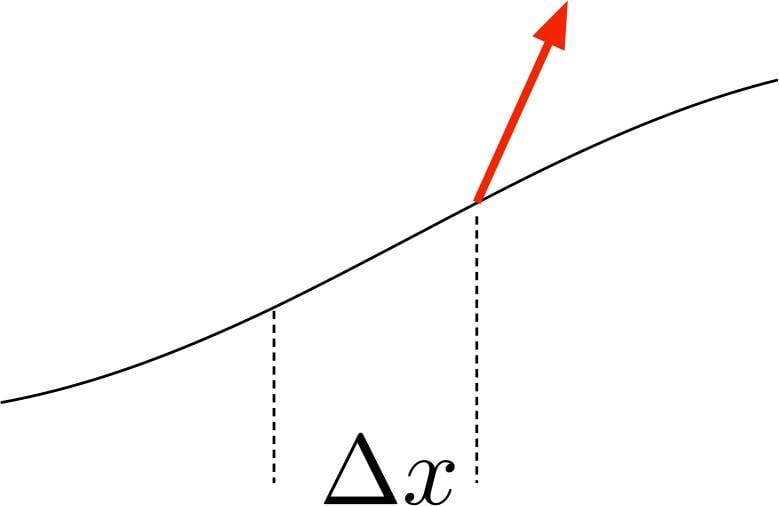vela said:
Which way do you think the force the righthand portion of the string exerts on the segment points? (That's why I asked you to draw in that force.) Do you think the situation I drew in the picture is possible?

View attachment 299851
No, I know that. You're not understanding my question. I attached another image. The blue arrow is one possibility of a resultant force vector in which ##\tan(\theta_2) = \frac{Fy}{Fx}##. The orange arrow is another possibility of a resultant force vectors in which ##\tan(\theta_3) = \frac{Fy}{Fx}##. ##\theta_2 != \theta_3##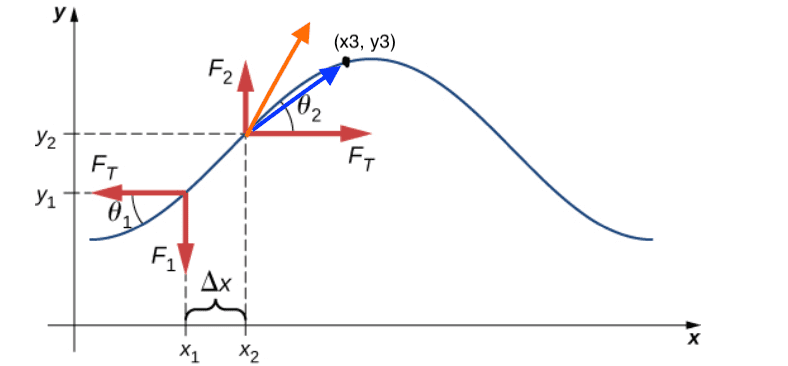You're right. I don't understand what you're asking. What do you mean by ##F_x## and ##F_y##? It's apparently not what I think you mean.

vela said:
You're right. I don't understand what you're asking. What do you mean by ##F_x## and ##F_y##? It's apparently not what I think you mean.
##F_x = F_T## and ##F_y = F_2##

Does this figure help?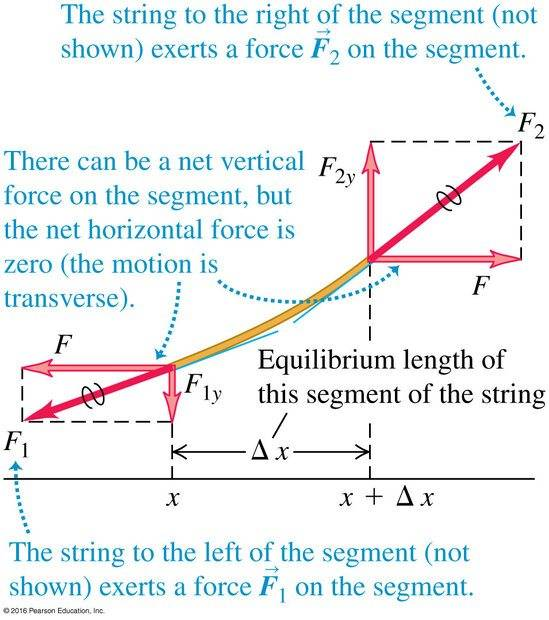vela said:
Does this figure help?

View attachment 299860
That's assuming that the string moves in the direction of the applied force

I have no idea why you think that.

annamal said:
No, I know that. You're not understanding my question. I attached another image. The blue arrow is one possibility of a resultant force vector in which ##\tan(\theta_2) = \frac{Fy}{Fx}##. The orange arrow is another possibility of a resultant force vectors in which ##\tan(\theta_3) = \frac{Fy}{Fx}##. ##\theta_2 != \theta_3##
View attachment 299857
The force on each point on the string can only come from adjacent parts of the string. Therefore, the force is always parallel to the string at each point. There is only one possibility for the angle, which is the angle the string itself makes.

DrClaude said:
The force on each point on the string can only come from adjacent parts of the string. Therefore, the force is always parallel to the string at each point. There is only one possibility for the angle, which is the angle the string itself makes.
Can you explain what you mean by adjacent parts?

annamal said:
Can you explain what you mean by adjacent parts?
Imagine the string as a large number of finite segments. The force on each segment is applied by the two adjacent segments, one on either side.

The continuous solution comes from taking the limit as the segment size tends to zero.

annamal said:
That's not answering my question of how come θ2 and θ1 are the angles between the forces.
Quite arbitrarily (but obviously the best choice) the tension force has been resolved into a lateral and longitudinal force. This sort of approach is the way we solve hundreds of problems but is probably only valid for small displacements and the diagram exaggerates the scale so that the vectors can be drawn on a diagram.

Sorry for starting at the bottom of this problem but it can often be worth while stating all the basics first.

As I was composing another reply, I think I figured out what your question was. Say you have a mass suspended by a string and you pull on the string to accelerate the mass to the right. I think you're envisioning a force on the mass like this: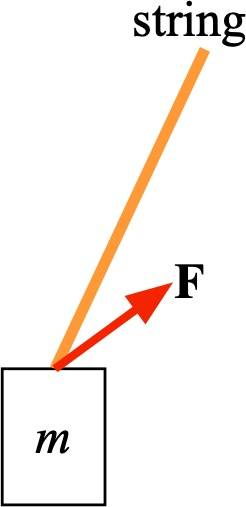where the force exerted by the string doesn't necessarily point in the same direction as the string.

In the typical model of a string, this won't happen. If the string were able to pull on the mass like that, Newton's third law says the mass would pull on the string with an equal and opposite force. The reaction force would causing the tilt of the string to increase, i.e. the string will rotate, until the force is collinear with the string. The assumption is that the string will respond so quickly that the force always along the direction of the string.

•sophiecentaur
PeroK said:
Imagine the string as a large number of finite segments. The force on each segment is applied by the two adjacent segments, one on either side.

The continuous solution comes from taking the limit as the segment size tends to zero.
What do you mean by adjacent segments? The x and y components to the net force?

annamal said:
What do you mean by adjacent segments? The x and y components to the net force?
No, a segment is part of the string. The diagram in your original post shows the segment between ##x_1## and ##x_2##.

The string segments exert forces on each other, like links in a chain.

•Ibix
Consider a series of beads of mass ##m## connected by massless rigid rods. With ##N=2## beads, it would look like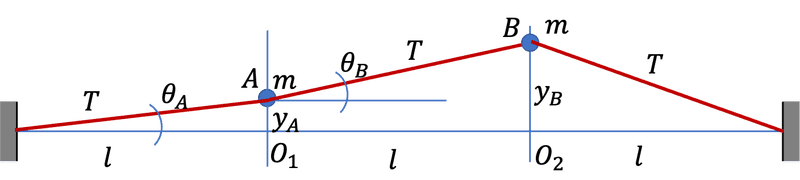You can consider a string as the limit of this model when ##N\rightarrow\infty## and ##l\rightarrow0##.

•vanhees71 and PeroK